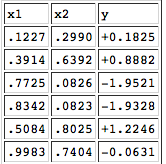# 315-ps1

Assignment #1

Due on Github 23:59 Sunday 13 August

Goal

The goal of this assignment is to introduce neural networks in terms of more basic ideas: linear regression and linear-threshold classification.

Part 0:  NumPy warmup

If you’re already familiar with NumPy, the numerical package we’ll use throughout this course, you can probably skip ahead to Part 1. Otherwise, fire up IDLE3 or your favorite Python3 interpreter, and do this:

    >>> import numpy as np


Now we can just use the abbreviation np whenever we want to use something from NumPy. For example, we can create an empty array like this:

    >>> x = np.array([])


To append a value v to the array:

    >>> x = np.append(x, v)


The power of NumPy is its ability to perform a single operation on an entire array at once. For example:

    >>> sum(x*x)


will return the sum of all the squared values in array x.  NumPy also adds the power of types (as in Java or C++) to your computations. The following code shows how you can use types to switch back and forth between boolean (True/False) and numerical (1/0) arrays:

     >>> model    = np.array([5, 1, 2, 3, 4])
>>> obtained = np.array([5, 6, 2, 3, 2])
>>> model == obtained
array([ True, False,  True,  True, False], dtype=bool)
>>> sum((model == obtained).astype('int')) / len(model)
0.59999999999999998


Here we see that our obtained values are in 60% agreement with the values from our model, with the extra digits due to the inevitable rounding error. Using this information and your existing knowledge of Python, you should be able to complete this assignment without too much difficulty.

Part 1

Consider the following table that describes a relationship between two input variables x1x2 and an output variable y.This is part of a larger data set that Prof. Michael Mozer created, which you can download in text format.  Using Python and NumPy, write a program to read in the data file and find the individual least squares solutions to y=mx1+by=mx1+b and y=mx2+by=mx2+b. You can use the formulas from the lecture slides. Don’t modify the data file, because I will use the original version when testing your code.

Part 2

Now solve the full linear regression y=w1x1+w2x2+by=w1x1+w2x2+b using np.linalg.lstsq. Hint: The example they give can be modified slightly to do what we need.  You should pass x1, x2 to np.vstack instead of just x. The output of np.linalg.lstsq(A, y) will then be your w1, w2, b instead of the m, c in their example.

Part 3

Turn this data set from a regression problem into a classification problem by running each pair of points x1,x2x1,x2 through the regression equation. In other words, use w1x1+w2x2+b>0w1x1+w2x2+b>0 as a criterion for assigning each point x1,x2x1,x2 to one class or the other. You can then compare this classification to the values in the zz column of the dataset. Report your success rate as a percentage. If you do this right, your solution to Part 3 will require only a couple of lines of code added to the code you wrote for Part 2.

Part 4

In machine learning, we really want to train a model based on some data and then expect the model to do well on “out of sample” data. Try this with the code you wrote for Part 3: Train the model on the first {25, 50, 75} examples in the data set and test the model on the final {75, 50, 25} examples, reporting percentage correct for each size. As a baseline test, do a final report on percentage correct when w1=w2=b=0w1=w2=b=0.

Part 5 (Extra credit)

Use matplotlib.pyplot to create figures for your linear regression models, similar to the ones in the lectures slides.

What to turn in

Put all your code in a module regression.py that I can load into idle and test by hitting F5. Your module should print out the results for each part of the assignment in a way that is easy to read. On this assignment and all assignments in this course, you will get a zero if I hit F5 and get an error.  No partial credit, no resubmission, no exceptions.